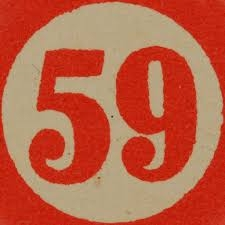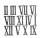# The larger

The larger of two numbers is nine more than four times the smaller number. The sum of the two numbers is fifty-nine. Find the two numbers.

a =  49
b =  10

### Step-by-step explanation:

a = 9 + 4b
a+b = 59

a = 9 + 4•b
a+b = 59

a-4b = 9
a+b = 59

a = 49
b = 10

Our linear equations calculator calculates it.Did you find an error or inaccuracy? Feel free to write us. Thank you!Tips to related online calculators
Do you have a linear equation or system of equations and looking for its solution? Or do you have a quadratic equation?

## Related math problems and questions:

• The sum 8The sum of two numbers is 21. If three times the smaller numbers is two less than twice the larger number, find the two numbers.
• The difference 2The difference between the two numbers is 25. The smaller number is 1/6th of the larger number. What is the value of the smaller number?
• The sum 16The sum of A and B is 36. The difference between A & B is 8. find the larger number of A.
• Sum of three numbersThe sum of three numbers from which the second number is 20% smaller than the first number and the third number is 25% smaller than the second number is 96. Determine these numbers.
• Two numbersOne number is by 79 larger than the other. If we divide the larger number by the smaller one, we get the ratio 5 and the remainder 11. Determine both numbers.
• Three numbersFind three numbers so that the second number is 4 times greater than the first and the third is lower by 5 than the second number. Their sum is 67.
• Two numbersThe difference between the two numbers is 74. If we divide a larger number by a smaller one, we get a quotient 7 and the rest of 2. Determine both numbers.
• Two numbersThe sum of the two numbers is 1. Find both numbers if you know that half of the first is equal to one-seventh of the second number.
• Ratio of two unknown numbersTwo numbers are given. Their sum is 30. We calculate one-sixth of a larger number and add to both numbers. So we get new numbers whose ratio is 5:7. Which two numbers were given?
• Two unknown numbersOne number is 2 less than a second number. Twice the second number is 14 less than 5 times the first. Find the two numbers.
• Unknown mixed numberFind the number that is smaller than 5 5/12 by as much as 2 2/13 is smaller than 6 1/6
• Four numbersThe first number is 50% second, the second number is 40% third, the third number is 20% of the fourth. The sum is 396. What are the numbers?
• Three figures - numbersThe sum of three numbers, if each is 10% larger than the previous one, is 662. Determine the figures.
• A number 4A number is made up of two digits. The sum of the digits is 11. if the digits are interchanged, the original number is increase by 9. Find the original number
• Digit sumThe digit sum of the two-digit number is nine. When we turn figures and multiply by the original two-digit number, we get the number 2430. What is the original two-digit number?
• Two numbers 7The sum of two consecutive even numbers is 30. Find the numbers.
• IntegerFind the integer whose distance on the numerical axis from number 1 is two times smaller as the distance from number 6.